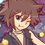# Geometry (1st math Thailand POSN 2014)

1.) Explain how to construct a square with the same area of any given quadrilaterals.

2.) Let $$P$$ be a point in $$\triangle ABC$$. Extend $$\overline{AP}, \overline{BP}, \overline{CP}$$ intersect $$\overline{BC}, \overline{CA}, \overline{AB}$$ at point $$D,E,F$$ respectively. If $$\square ACDF$$ and $$\square BCEF$$ are cyclic quadrilaterals, prove that $$\square PDBF$$ is also cyclic quadrilateral.

3.) Use Ceva's theorem to prove that the 3 angle bisectors of $\triangle ABC$ intersect at 1 point.

4.) Let $\triangle ABC$ with point $I$ as incenter. Extend $\overline{AI}$ intersect circumcircle of $\triangle ABC$ at point $P$.

• 4.1) If point $I_{a}$ is the center of excircle of $\triangle ABC$ which is opposite to angle $A$, prove that $P$ is the center of the circumcenter of $\square ICI_{a}B$

• 4.2) Prove that $\displaystyle 2\sin{(B\hat{I}C)} = \frac{BC}{PI}$

5.) Let $\square PQRS$ has an incircle, which is tangent to $\overline{SP}, \overline{PQ}, \overline{QR}, \overline{RS}$ at point $A,B,C,D$ respectively. Extend $\overline{AB}$ intersect $\overline{SQ}$ at point $X$. Prove that point $C,D,X$ are collinear.

This is the part of Thailand 1st round math POSN problems.Note by Samuraiwarm Tsunayoshi
6 years, 9 months ago

This discussion board is a place to discuss our Daily Challenges and the math and science related to those challenges. Explanations are more than just a solution — they should explain the steps and thinking strategies that you used to obtain the solution. Comments should further the discussion of math and science.

When posting on Brilliant:

• Use the emojis to react to an explanation, whether you're congratulating a job well done , or just really confused .
• Ask specific questions about the challenge or the steps in somebody's explanation. Well-posed questions can add a lot to the discussion, but posting "I don't understand!" doesn't help anyone.
• Try to contribute something new to the discussion, whether it is an extension, generalization or other idea related to the challenge.

MarkdownAppears as
*italics* or _italics_ italics
**bold** or __bold__ bold
- bulleted- list
• bulleted
• list
1. numbered2. list
1. numbered
2. list
Note: you must add a full line of space before and after lists for them to show up correctly
paragraph 1paragraph 2

paragraph 1

paragraph 2

[example link](https://brilliant.org)example link
> This is a quote
This is a quote
    # I indented these lines
# 4 spaces, and now they show
# up as a code block.

print "hello world"
# I indented these lines
# 4 spaces, and now they show
# up as a code block.

print "hello world"
MathAppears as
Remember to wrap math in $$ ... $$ or $ ... $ to ensure proper formatting.
2 \times 3 $2 \times 3$
2^{34} $2^{34}$
a_{i-1} $a_{i-1}$
\frac{2}{3} $\frac{2}{3}$
\sqrt{2} $\sqrt{2}$
\sum_{i=1}^3 $\sum_{i=1}^3$
\sin \theta $\sin \theta$
\boxed{123} $\boxed{123}$

Sort by:

1.) I tried to make the rectangle, let's say length $a,b$. I tried to do by making a line length $a+b$ and draw a semicircle diameter of $a+b$. Then I draw a perpendicular line at where the sides join and we get length $\sqrt{ab}$. And we're done, maybe.

- 6 years, 9 months ago

2) $\angle FDP=\angle ADF=\angle ACF$ since $ACDF$ is cyclic. $\angle ACF=\angle ECP=\angle FBP$ since $BCEF$ is cyclic. Hence $\angle FBP=\angle FDP \implies PDBF$ is cyclic.

- 6 years, 9 months ago

Problem 1: Easy one. See here.

Problem 2: First notice that $\angle PEF=\angle BEF=\angle BCF=\angle DCF=\angle DAF=\angle PAF$ so $AFPE$ is cyclic. Hence $\angle DBF=\angle CBF=\angle AEF=\angle APF=\pi-\angle FPD$ implies $PDBF$ is cyclic. Furthermore notice that $\angle PDB=\angle ADB=\pi-\angle ADC=\pi-\angle AFC=\angle BFP$ so $\angle ADB=\angle CFB=90^\circ$. So $P$ must be the orthocenter of $\triangle ABC$.

Problem 3: Consider $\triangle ABC$ where $AD$, $BE$, $CF$ are angle bisectors, with $D,E,F$ intersections with opposite sides. By Angle Bisector Theorem we have $BD/DC=AB/AC$, $CE/EA=BC/AB$, $AF/FB=AC/BC$. Multiplying these cancels Ceva. $\square$

Problem 4: Check the problem, the wordings are wrong.

Problem 5: Menelaus on $\triangle QRS$.

- 6 years, 9 months ago

No.4: Try right-click and open this image in new tab. I checked the problem 4 times and didn't see what is wrong.alt text

- 6 years, 8 months ago